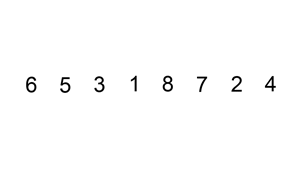🧠

# Insertion Sort• Builds sorted list one item at a time
• Less efficient on large lists compared to other algorithms

## Complexity

Average Time Space
$O(n^2)$ $1$

## Process

• Keep front of the list sorted
• Loop through unsorted elements
• Place them in proper position in sorted elements

## Implementations

### JavaScript

function insertionSort(array) {
// Loop through all elements in the array
for (let i = 0; i < array.length; i++) {
let currIndex = i;

// If the previous element is greater than the current we swap
while(array[currIndex - 1] !== undefined && array[currIndex] < array[currIndex - 1]) {
// Swap the elements
[array[currIndex - 1], array[currIndex]] = [array[currIndex], array[currIndex - 1]];

// Shift the current index back
currIndex--;
}
}

return array;
}